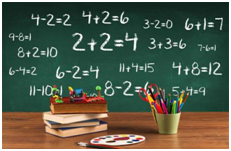(1)判断几个项是否是同类项有两个条件：

①所含字母相同；

②相同字母的指数分别相等，同时具备这两个条件的项是同类项，缺一不可．

(2)同类项与系数无关，与字母的排列顺序无关．

(3)一个项的同类项有无数个，其本身也是它的同类项．

1. 概念：把多项式中的同类项合并成一项，叫做合并同类项．

2．法则：合并同类项后，所得项的系数是合并前各同类项的系数的和，且字母部分不变．

(1)不是同类项的不能合并，无同类项的项不能遗漏,在每步运算中照抄．

(2)系数相加(减)，字母部分不变，不能把字母的指数也相加(减)．

（1）如果两个同类项的系数互为相反数，合并同类项后，结果为0。

（2）不要漏掉不能合并的项。

（3）只要不再有同类项，就是结果（可能是单项式，也可能是多项式）。

（4）不是同类项千万不能进行合并。

1.计算a^2+3a^2的结果是(    )

A.3a^2B.4a^2C.3a^4D.4a^

2.下面运算正确的是(    ).

A.3a+2b=5ab

B.a^2b-3ba^2=0

C.3x^2+2x^3=5x^5

D.3y^2-2y^2=1

3.下列计算中,正确的是(     )

A、2a+3b=5ab

B、a3-a2=a

C、a2+2a2=3a2

D、(a-1)0=1.

4.已知一个多项式与3x^2+9x的和等于3x^2+4x-1,则这个多项式是(    )

A.-5x-1B.5x+1C.-13x-1D.13x+1

5.下列合并同类项正确的是

A.2x+4x=8x^2

B.3x+2y=5xy

C.7x^2-3x^2=4

D.9a^2b-9ba^2=0

6.加上-2a-7等于3a^2+a的多项式是（ ）

A.3a^2+3a-7

B.3a^2+3a+7.

C.3a^2-a-7

D.-4a^2-3a-7

7.a=1,a-2a+3a-4a+......+99a-100a的值为（

A.5050      B.100      C.50      D.-50

12(2a^2+9b)+3(-5a^2-4b)

23x^2+2xy-4y^2-3xy+4y^2-3x^2

1、解：原式=4a^2+18b-15a^2-12b=-11a^2+6b

2、解：原式=3x^2-3x^2+(2xy-3xy)+(4y^2-4y^2)=-xy